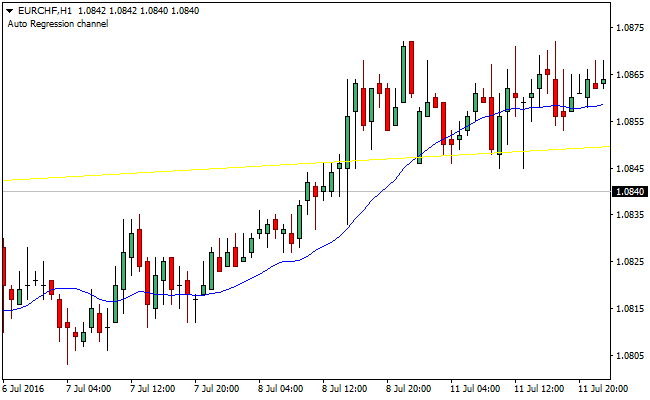# Regression Channel MT4 Forex IndicatorThe regression channel indicator for MetaTrader 4 is one that draws a linear regression channel. The indicator starts off by drawing a trend line between two dots, then on that foundation it draws a channel of two parallel lines, which will be placed at the same distance from the regression line.

It is the value of the maximum deviation of the closing price from the mean line that determines the distance to the regression line. If price test the boundary of the channel, or possibly breaks it, then it is an indication of an incoming trend reversal.

Example ChartThe regression_channel indicator plots a linear regression channel unto the trading chart, which in turn implies the plotting of channels to best align with the price. There are three components of the regression_channel indicator:

The linear Regression Line: This is a line that is most suitable to all the data point of importance.

The Upper Channel Line: The upper channel line is drawn parallel to the linear regression line and is in most cases one or two standard deviations above the linear regression line.

The Lower Channel Line: The lower channel line is drawn below the linear regression line running parallel to it and it is in most cases also one or two standard deviations below the linear regression line.

A trader might expect a bullish trend or signal when price falls below the lower channel line, while a selling opportunity might present itself when price breaks above the upper channel line. Howbeit, there’s need to use other technical indicator tools to confirm further.

MT4 Indicator Characteristics

Currency pairs: Any